# International Economics Study Set 8

## Quiz 8 :The Instruments of Trade PolicyLooking for Economics Homework Help?

## Quiz 8 :The Instruments of Trade PolicyHome's demand curve for wheat is D = 100 ? 20 P. Its supply curve is S = 20 + 20 P. Derive and graph Home's import demand schedule. What would the price of wheat be in the absence of trade?
Free
Essay

Home import demand is the excess of demand of Home consumers over the supply of Home producers. So, Home import demand ( MD )equation is obtained by the subtraction of Home producers supply ( S )from home consumers demand ( D ).
MD = D - S = (100 - 20 P )- (20+20 P )= 100 -20 P - 20 - 20 P = 80 -40 P...... (1)In the absence of trade, domestic demand and domestic supply equals each other such that import demand is zero. Thus, the price of wheat in the Home country in the absence of trade is
M D = 80 - 40 P
0 = 80 - 40
40 P = 80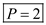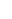If the price of wheat increases, Home consumers demand less, but Home producers supply more, so, import demand declines. This inverse relationship between the price of the good and its demand for imports when depicted graphically gives rise to a downward sloping import demand curve which is show in the diagram below: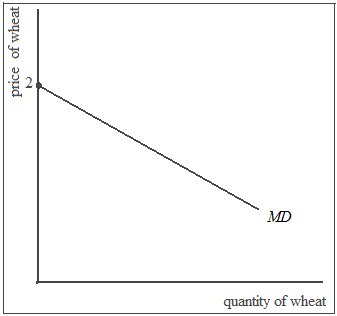Import Demand Curve
At price 2 the MD curve intercepts the price axis (import demand = 0 at price 2)Now add Foreign, which has a demand curve D * = 80 ? 20 P and a supply curve S * = 40 + 20 P. a. Derive and graph Foreign's export supply curve and find the price of wheat that would prevail in Foreign in the absence of trade. b. Now allow Foreign and Home to trade with each other, at zero transportation cost. Find and graph the equilibrium under free trade. What is the world price? What is the volume of trade?
Free

There is no answer for this questionHome imposes a specific tariff of 0.5 on wheat imports. a. Determine and graph the effects of the tariff on the following: (1) the price of wheat in each country; (2) the quantity of wheat supplied and demanded in each country; (3) the volume of trade. b. Determine the effect of the tariff on the welfare of each of the following groups: (1) Home import-competing producers; (2) Home consumers; (3) the Home government. c. Show graphically and calculate the terms of trade gain, the efficiency loss, and the total effect on welfare of the tariff.
Free
Essay

a)In the absence of tariff, the price of wheat is 1.5 in both Home and Foreign.
Given:
• Home's demand curve for wheat is:
o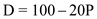• Home's supply curve for wheat is:
o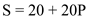• Foreign demand curve for wheat:
o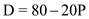• Foreign supply curve for wheat is:
o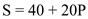With the imposition of tariff of 0.5 by the Home country, shippers will be unwilling to move the wheat from Foreign to Home unless the Home price exceeds the foreign price by at least the tariff rate of 0.5.
However, there will be an excess demand for wheat in Home and an excess supply in Foreign, if there is no shipping of wheat.
Thus, the price in Home will rise and that in Foreign will fall until the price difference is 0.5.
Therefore, a tariff drives a wedge between the prices in the two markets.
The equation for new MD curve is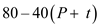Where:
• P is the price, equal to 1.5, and
• t is the tariff rate, equal to 0.5.
The equation for new XS curve is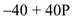The equation for the export supply curve by the foreign country is unchanged. Setting the import demand ( MD )equal to export supply ( XS )we solve the value of P as:Therefore, the price of wheat in foreign country is 1.25.
Now, adding the new price with the tariff rate gives us the domestic price as:Therefore, the domestic price is 1.75.
Thus, to summarize:
• The world price is 1.25
• The price in home country is 1.75
• The price in foreign country is 1.25
Given:
Home's demand curve for wheat is:Home's supply curve for wheat is:Now, substitute the above-calculated prices in the equations for demand and supply to get the demand and supply for Home and Foreign.
Accordingly,Similarly,Now, calculate the volume of trade by the equation for MD or XS :Therefore, the volume of trade is 10.
The diagram below shows the effect of tariff on the trade market: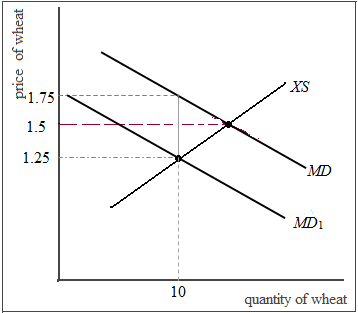Effect of Tariff on Trade Market
In the above diagram, the intersection of MD curve and XS curve determine the world price for wheat at 1.5.
With the imposition of tariff, the MD curve shifts to MD 1.
After tariff, the home price increases to1.75 while the foreign price falls to 1.25 so that the difference between the two is equal to the amount of tariff.
In Home, imports will be fewer as producers supply more at a higher price, while consumers demand less.
In Foreign, lower price leads to reduced supply and increased demand. Thus, export supply is smaller and the volume of wheat traded declines from 20 to 10.
b)(1)Home import-competing producers are better off because they get higher prices for their goods and that induces them to increase the supply of wheat.
Moreover, they have less competition from the foreign producers.
(2)Home consumers are worse off because they have to pay a higher price for wheat.
(3)The Home government benefits because now they have additional tariff revenue.
c)The following diagram shows the terms of trade gain, the efficiency loss, and the total effect on welfare of the tariff: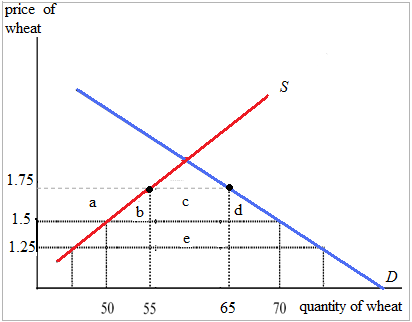Effect of Tariff on the Trade Gain, Efficiency Loss Welfare
The cost and benefits of a tariff for the importing country is shown by the five area a , b , c , d and e. Let us first calculate the values for the following areas: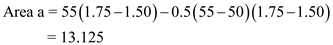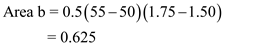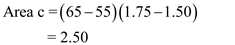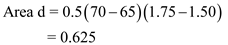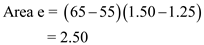Note: In the calculation of area a , b , and c which are triangles, the formula is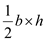.
As a tariff lowers foreign export price, trade gains arise. Rectangle e in the above diagram represents the trade gain.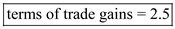In the above diagram, triangles b + d represent the efficiency loss that arises because of tariff distorts.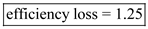The welfare effect of tariff on the three players of the economy is as follows: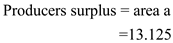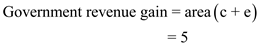Suppose Foreign had been a much larger country, with domestic demand D * = 800 ? 200 P , S * = 400 + 200 P. (Notice that this implies the Foreign price of wheat in the absence of trade would have been the same as in problem ) Recalculate the free trade equilibrium and the effects of a 0.5 specific tariff by Home. Relate the difference in results to the discussion of the small country case in the text. Problem Now add Foreign, which has a demand curve D * = 80 ? 20 P and a supply curve S * = 40 + 20 P. a. Derive and graph Foreign's export supply curve and find the price of wheat that would prevail in Foreign in the absence of trade. b. Now allow Foreign and Home to trade with each other, at zero transportation cost. Find and graph the equilibrium under free trade. What is the world price? What is the volume of trade?
EssayWhat would be the effective rate of protection on bicycles in China if China places a 50 percent tariff on bicycles, which have a world price of $200, and no tariff on bike components, which together have a world price of$100?
EssayThe United States simultaneously limits imports of ethanol for fuel purposes and provides incentives for the use of ethanol in gasoline, which raise the price of ethanol by about 15 percent relative to what it would be otherwise. We do, however, have free trade in corn, which is fermented and distilled to make ethanol, and accounts for approximately 55 percent of its cost. What is the effective rate of protection on the process of turning corn into ethanol?
EssayReturn to the example of problem. Starting from free trade, assume that Foreign offers exporters a subsidy of 0.5 per unit. Calculate the effects on the price in each country and on welfare, both of individual groups and of the economy as a whole, in both countries. Problem Now add Foreign, which has a demand curve D * = 80 ? 20 P and a supply curve S * = 40 + 20 P. a. Derive and graph Foreign's export supply curve and find the price of wheat that would prevail in Foreign in the absence of trade. b. Now allow Foreign and Home to trade with each other, at zero transportation cost. Find and graph the equilibrium under free trade. What is the world price? What is the volume of trade?
EssayUse your knowledge about trade policy to evaluate each of the following statements: a. "An excellent way to reduce unemployment is to enact tariffs on imported goods." b. "Tariffs have a more negative effect on welfare in large countries than in small countries." c. "Automobile manufacturing jobs are heading to Mexico because wages are so much lower there than they are in the United States. As a result, we should implement tariffs on automobiles equal to the difference between U.S. and Mexican wage rates."
Essay